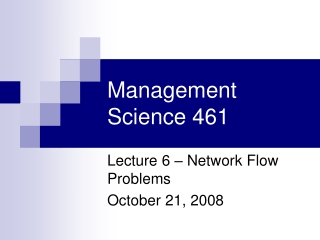DownloadDownload PresentationManagement Science 461

# Management Science 461

Télécharger la présentation## Management Science 461

- - - - - - - - - - - - - - - - - - - - - - - - - - - E N D - - - - - - - - - - - - - - - - - - - - - - - - - - -
##### Presentation Transcript

1. Management Science 461 Lecture 6 – Network Flow Problems October 21, 2008

2. Intro • Go back to Red Dog • Show how a number of DM models and techniques are special cases of • Min-cost network flow problem

3. Example from MGTSC 352 Each arc has positive cost Supply nodes have negative values; demand nodes are positive Transshipment nodes have 0 demand, 0 supply Capacity of 10 per arc This is a balanced problem – why?

4. Model • Define Xij = amount of product from node i to node j • Objective Function: minimize total cost

5. Model • For each node, we have a flow conservation constraint Incoming (Inflow) In + Supply = Out + Demand In – Out = Demand – Supply In – Out ≥ Demand – Supply Supply Demand Outgoing (Outflow)

6. All Constraints

7. New model • Constraints hard to write out – but there’s an easier way. • Node Arc Incidence Matrix cleans up this process but also reveals the structure of the problem • Every row corresponds to an arc, and has at most one “1” and one “-1” entry • Therefore, matrix is unimodular

8. Unimodularity • References for all your unimodular needs: • Nemhauser & Wolsey, Integer & Combinatorial Optimization • Ahuja, Magnanti, and Orlin, Network Flows • Unimodular matrix: “a nonsingular matrix with a determinate of ±1; a square matrix is totally unimodular if every nonsingular submatrix from it is unimodular.” So there.

9. Why do we care? • Integer solutions without having to specify integer values • Integer constraints force more costly Solver computation • So important that other problems are modeled as transportation problems e.g. inventory

10. Summary • Min cost network flow: • Objective function: min cost • Capacity on arcs • Flow conservation • Special Cases: • Shortest path (source and sink nodes, no capacities) • Transshipment: No capacities • Transportation: All nodes either suppy or demand • Max flow problems – identify capacity constraints

11. Add location to the mix • This is what you did in Red Dog, MGTSC 352 • Minimize fixed cost of location + transportation cost • Constraints as before (flow conservation and capacity) • Add binary variables for facility location

12. Effects on flow conservation • Add binary Yj as follows: • Sumproduct in objective function (fixed cost) • New flow balance constraint becomesIN – OUT ≥ DEMAND – SUPPLY*Y • If Y = 0, make sure we satisfy demand (positive transportation costs ensure we never “pool” product at the node) • If Y=1, we have the constraint as before, ensures we produce enough and ship it out.